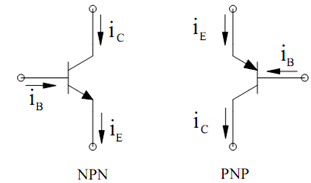## Bipolar Junction Transistor Assignment Help

Assignment Help: >> Transistor Amplifier Circuits - Bipolar Junction Transistor

Bipolar Junction Transistor:

The notations utilized here for voltages and currents correspond to the following conventions : DC bias values are mentioned by an upper case letter with upper case subscripts, for example IC , VDS. Instantaneous values of small-signal variables are mentioned by a lower-case letter with lower-case subscripts, e.g. υs, ic. Total values are indicated by a lower-case letter with upper-case subscripts, foe instance. υBE, iD. Circuit symbols for independent source are circular and symbols for controlled sources have a diamond shape. Voltage sources have a ± sign within the symbol and current sources have an arrow.

Figure illustrated the circuit symbols for the NPN and PNP BJTs. The collector-base junction is reverse-biased and the base-emitter junction is forward biased in the active mode. For the NPN device, the active-mode collector and base currents are specified by

iC  = I exp(υ BE /VT) ; iB  =   iC / βFigure: BJT Circuit Symbols

where VT   refer to the thermal voltage, IS refer to the saturation current, and β is refers to the current gain. These are given by

VT  = kT/ q = 0.025 V  for T = 290; K = 25.86 m V for T = 300 K

IS   = ISOCE /VA)

and

β= β0 (1 +  (υCE /VA ))

where VA is the early voltage and ISO and βo, respectively, are the zero values of IS and β.

Because  I S/ β = ISO/ β0 , it follows that i  is not a function of υCE. The equations apply to the pnp device if the subscripts BE and CE are reversed.

The emitter-to-collector current gain α is defined as the ratio iC / iE .To solve for this, we

may write

i E  = i B   + i C = ((1/ β )+ 1)  i C = (1 + β/ β) i C

It follows that

α= iC/ iE  =        β/1 + β

Therefore, the three transistor currents are associated by the equation

iC  =β iB  = α iE